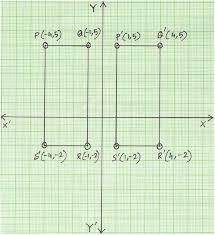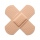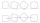# Area and perimeter of rectangle

The content area of the rectangle is 3000 cm2, one dimension is 10 cm larger than the other. Determine the perimeter of the rectangle.

o =  220 cm

### Step-by-step explanation:

Our quadratic equation calculator calculates it.Did you find an error or inaccuracy? Feel free to write us. Thank you!Tips to related online calculators
Looking for help with calculating roots of a quadratic equation?
Do you have a linear equation or system of equations and looking for its solution? Or do you have a quadratic equation?

#### You need to know the following knowledge to solve this word math problem:

We encourage you to watch this tutorial video on this math problem:

## Related math problems and questions:

• Square and rectangleCalculate the side of a square which content area equals area of the rectangle having a length of 3 cm greater and by 2 cm smaller than the side of the square.
• Dimensions of the trapezoidOne of the bases of the trapezoid is one-fifth larger than its height, the second base is 1 cm larger than its height. Find the dimensions of the trapezoid if its area is 115 cm2
• Rectangle diagonalsIt is given a rectangle with an area of 24 cm2 a circumference of 20 cm. The length of one side is 2 cm larger than the length of the second side. Calculate the length of the diagonal. Length and width are yet expressed in natural numbers.
• RectangleThe rectangle has a perimeter 75 cm. Diagonal length is 32.5 cm. Determine the length of the sides.
• Two patchesPeter taped the wound with two rectangular patches (one over the other to form the letter X). The area sealed with both patches at the same time had a content of 40cm2 and a circumference of 30cm. One of the patches was 8cm wide. What was the width of the
• The poolThe cube-shaped pool has 140 cubic meters of water. Determine the dimensions of the bottom if the depth of the water is 200 cm and one dimension of the base is 3 m greater than the other. What are the dimensions of the pool bottom?
• RectangleThe perimeter of the rectangle is 22 cm and content area 30 cm2. Determine its dimensions, if the length of the sides of the rectangle in centimeters is expressed by integers.
• Rectangle vs squareOne side of the rectangle is 1 cm shorter than the side of the square, the second side is 3 cm longer than the side of the square. Square and rectangle have the same content. Calculate the length of the sides of a square and a rectangle.
• Perimeter from areaWhat is the perimeter of the square if its content is 64 cm2?
• RectangleThe length of the rectangle is 12 cm greater than 3 times its width. What dimensions and area this rectangle has if ts circumference is 104 cm.
• RectangleThere is a rectangle with a length of 12 cm and a diagonal 8 cm longer than the width. Calculate the area of a rectangle.
• Perimeter and legsDetermine the perimeter of a right triangle if the length of one leg is 75% length of the second leg and its content area is 24 cm2.
• Second sideCalculate the length of the other side of the rectangle if its circumference is 60 cm and one side is 10 cm long.
• In theIn the rectangle ABCD, the distance of its center from the line AB is 3 cm greater than from the line BC. The circumference of the rectangle is 52 cm. Calculate the contents of the rectangle. Express the result in cm2.
• Diamond diagonalsCalculate the diamond's diagonal lengths if its content is 156 cm2 and the side length is 13 cm.
• Perimeter to area 2Find the area of a square whose perimeter is 100 cm with solve.
• Rectangle SSPerimeter of a rectangle is 268 cm and its diagonal is 99.3 cm. Determine the dimensions of the rectangle.